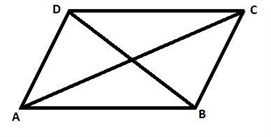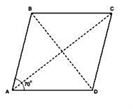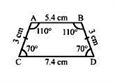RS Aggarwal Class 6 Solutions Chapter 17 - Quadrilaterals

RS Aggarwal Class 6 Chapter 17 - Quadrilaterals Solutions Free PDF

A quadrilateral is a polygon with four sides and four vertices. In this chapter, the students will learn about the different properties of quadrilaterals and various problems related to it. To understand the concepts of quadrilaterals students are advised to solve questions from RS Aggarwal for class 6. To help students we have also provided the RS Aggarwal class 6 solutions chapter 17 quadrilaterals.

Exercise 17A

Question 1:Name:

(i) its diagonals,

(ii) two pairs of opposite sides,

(iii) two pairs of opposite angles,

(iv) two pairs of adjacent sides,

(v) two pairs of adjacent angles.

Solution:

(i) The diagonals are AC and BD.

(ii) AB and CD, and AD and BC are the two pairs of opposite sides.

(iii) $\angle$A and $\angle$C, and $\angle$B and $\angle$D are the two pairs of opposite angles.

(iv) AB and BC, and AD and DC are the two pairs of adjacent sides.

(v) $\angle$A and $\angle$B, and $\angle$C and $\angle$D are the two pairs of adjacent angles.

Question 2:

Draw a parallelogram ABCD in which AB = 6.5 cm, AD = 4.8 cm and $\angle$BAD = 70o. Measure its diagonals.

Solution:

Since ABCD is a parallelogram, AB = DC = 6.5 cm and AD = BC = 4.8 cm.

Given:

$\angle$A = 70oSteps of construction:

1. Draw AD equal to 4.8 cm.

2. Make an angle of 70o at A and cut an arc of 6.5 cm. Name it B.

3. Cut an arc of 4.8 cm from B and 6.5 cm from D. Name it C.

4. Join AB, BC and CD.

5. Measuring the diagonal AC and BD, we get AC equal to 9.2 cm and BD equal to 6.6. cm.

Question 3:

Two sides of a parallelogram are in the ratio 4 : 3. If its perimeter is 56 cm, find the lengths of its sides.

Solution:

Two sides of a parallelogram are in the ratio 4 : 3.

Let the two sides be 4x and 3x.

In a parallelogram, opposite sides are equal and parallel. So, they are also in the ratio of 4 : 3, i.e. 4x and 3x.

Perimeter = 4x + 3x + 4x + 3x

$\Rightarrow$ 56 = 14 x

$\Rightarrow$ x = $\frac{56}{14}$

$\Rightarrow$ x = 4

Therefore, 4x = 16 and 3x = 12

Lengths of its sides are 16 cm, 12 cm, 16 cm and 12 cm.

Question 4:

Name each of the following parallelograms:

(i) The diagonals are equal and the adjacent sides are unequal.

(ii) The diagonals are equal and the adjacent sides are equal.

(iii) The diagonals are unequal and the adjacent sides are equal.

Solution:

(i) Rectangle

(ii) Square

(iii) Rhombus

Question 5:

What is a trapezium? When do you call a trapezium an isosceles trapezium? Draw an isosceles trapezium. Measure its side and angles.

Solution:A trapezium has only one pair of parallel sides.

A trapezium is said to be an isosceles trapezium if its non-parallel sides are equal.

Following are the measures of the isosceles trapezium:

AB = 5.4 cm

BC = 3 cm

DC = 7.4 cm

$\angle$A = $\angle$B = 110o

$\angle$D = $\angle$C = 70o

Question 6:

Which of the following statements are true and which are false?

(a) The diagonals of a parallelogram are equal.

(b) The diagonals of a rectangle are perpendicular to each other.

(c) The diagonals of a rhombus are equal.

Solution:

(a) False

(b) False

(c) False

Question 7:

Give reasons for the following:

(a) A square can be thought of as a special rectangle.

(b) A square can be thought of as a special rhombus.

(c) A rectangle can be thought of as a special parallelogram.

(d) A square is also a parallelogram.

Solution:

(a) This is because a rectangle with equal sides becomes a square.

(b) This is because a rhombus with each angle a right angle becomes a square.

(c) This is because a parallelogram with each angle a right angle becomes a rectangle.

(d) This is because in a square opposite side are parallel.

Question 8:

A figure is said to be regular if its sides are equal in length and angles are equal in measure. What do you mean by a regular quadrilateral?

Solution:

A square is a regular quadrilateral all of whose sides are equal in length and all whose angles are equal in measure.

Exercise 17B

OBJECTIVE QUESTIONS

Mark against the correct answer in each of the following:

Question 1:

The sum of all the angles of a quadrilateral is

(a) 180o

(b) 270o

(c) 360o

(d) 400o

Solution:

(c) 360o

The sum of all the angles of a quadrilateral is 360o.

Question 2:

The three angles of a quadrilateral are 80o, 70o and 120o. The fourth angle is

(a) 110o

(b) 100o

(c) 90o

(d) 80o

Solution:

(c) 90o

The three angles of a quadrilateral are 80o, 70o and 120o.

Let the fourth angle be x.

We know that the sum of all the angles of a quadrilateral is 360o.

80o + 70o + 120o + x = 360o

$\Rightarrow$ 270o + x = 360o

$\Rightarrow$ x = 360o – 270o

$\Rightarrow$ x = 90o

Thus, the fourth angle is 90o.

Question 3:

The angles of a quadrilateral are in ratio 3: 4: 5: 6. The largest of these angles is

(a) 90o

(b) 120o

(c) 150o

(d) 102o

Solution:

Let the angles of a quadrilateral be 3x, 4x, 5x and 6x.

Sum of all the angles of a quadrilateral is 360o.

Therefore, 3x + 4x + 5x + 6x = 360o

$\Rightarrow$ 18x = 360o

$\Rightarrow$ x = $\frac{360}{18}$

$\Rightarrow$ x = 20o

So,

3x = 60o

4x = 80o

5x = 100o

6x = 120o

The largest of these angles is 120o.

So, the correct answer is given in option (b).

Question 4:

A quadrilateral having one and only one pair of parallel sides is called

(a) a parallelogram

(b) a kite

(c) a rhombus

(d) a trapezium

Solution:

(d) a trapezium

A trapezium is a quadrilateral that has only one pair of parallel sides.

Question 5:

A quadrilateral whose opposite sides are parallel is called

(a) a rhombus

(b) a kite

(c) a trapezium

(d) a parallelogram

Solution:

(d) a parallelogram

A parallelogram is a quadrilateral whose opposites sides are parallel.

Question 6:

An isosceles trapezium has

(a) equal parallel sides

(b) equal non parallel sides

(c) equal opposite sides

(d) none of these

Solution:

(b) equal non parallel sides

The non-parallel sides of an isosceles trapezium are equal.

Question 7:

If the diagonals of a quadrilateral bisect each other at right angles, this quadrilateral is

(a) a rectangle

(b) a rhombus

(c) a kite

(d) none of these

Solution:

(b) a rhombus

The diagonals of a rhombus bisect each other at right angle.

Question 8:

A square has

(a) all sides equal and diagonals unequal

(b) all sides equal and diagonals equal

(c) all sides unequal and diagonals equal

(d) none of these

Solution:

(b) all sides equal and diagonals equal

In a square, all the sides are equal. All of its diagonals are also equal.

Question 9:

A quadrilateral having two pairs of equal adjacent sides but unequal opposite sides, is called a

(a) trapezium

(b) parallelogram

(c) kite

(d) rectangle

Solution:

(c) kite

A kite has two pairs of equal adjacent sides, but unequal opposite sides.

Question 10:

What do you mean by regular quadrilateral?

(a) A rectangle

(b) A rhombus

(c) A square

(d) A trapezium

Solution:

(c) A square

The only regular quadrilateral is a square. This is because of all its sides and angles are equal.

Practise This Question

Identify radius, centre, chord and diameter in the given circle.